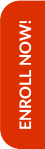Olympiads are the stepping stones to achieve better results in the competitive world that lies ahead in the life of the child. Math Olympiad examinations help students to improve their mathematical skills along with their analytical and problem solving abilities.

Hence, Olympiad Success Live has designed the course for Math Olympiad for class 5 in such a way that the foundation of the child is built up. For this, we have done great efforts in finding the tutor for class 5 Math Olympiad with relevant background and experience.

If you are interested in purchasing this course, then please Enrol Now. You will be redirected to the batch detail page, wherein you can see all the details like batch start and demo dates, fess and the registration link related to Math Olympiad for class 5 course.

##### Syllabus

Number Sense, Roman Numerals

• Number sense
• Natural number, whole number and integer
• Place and face value
• Successor and predecessor
• Rounding numbers (Up to 10,000)
• To write a numeral
• Roman numerals (Up to 1000)
• Indian system of numeration
• International system of numeration

Computation Operations

• Operations on large numbers
• Addition and subtraction of 6-digits numbers
• Word problems on addition and subtraction
• Multiplication and division of large numbers
• Word problems on subtraction and division
• Simplification
• Numerical expressions
• BODMAS rule

Fractions

• Factors and multiples
• Definition and properties of factor and multiples
• Even and odd numbers
• Prime and composite numbers
• Prime factorization
• HCF and LCM
• Fraction
• Definition of fraction
• Various types of fractions (Like, unlike, unit, proper, improper, mixed and equivalent fraction)
• To convert an improper fraction into a mixed fraction
• To convert a mixed number into an improper fraction
• Lowest form of fraction
• Operations of fraction (Addition, subtraction, multiplication and division)
• Comparison of fractions
• Playing with numbers
• Test of divisibility by 2, 3, 4, 5, 6, 7, 8, 9, 10 and 11

Measurements

• Measures of length
• Conversion of higher units into lower unit and vice versa (mm-cm-dm-m-dam-hm-km)
• Addition and subtraction of length measures
• Multiplication and division of length measures
• Measures of mass
• Conversion of higher units into lower unit and vice versa (kg-hg-dag-g-dg-cg-mg)
• Addition and subtraction of mass measures
• Multiplication and division of mass measures
• Measures of capacity
• Conversion of higher units of volume into lower unit and vice versa (kl-hl-dal-l-dl-cl-ml)
• Addition and subtraction of capacity measures
• Multiplication and division of capacity measures

Temperature

• Body temperature
• Converting from Celsius to Fahrenheit and vice versa

Time

• Time
• To convert 24-hour clock time to 12-hour clock time
• Addition and subtraction of time
• Duration of an activity
• Money
• Conversion of rupees into paise and vice versa
• Operations of money (Addition, subtraction, multiplication and division of money)

Basic Geometrical Concepts

• Concept of angles
• Definition of plane, point, line segment, line and ray
• Definition and comparison of angles
• Classification of angles
• Complementary and supplementary angle
• Parallel and perpendicular lines
• Triangles
• Collinear and non-collinear points
• Classification of triangles (According to their sides and angles)
• Introduction to circle
• Terms related to circle
• Perimeter and Area
• Perimeter and area of rectangle
• Perimeter and area of square
• Perimeter and area of triangle
• Volume
• Volume of cube and cuboid
• Units of volume

Symmetry and Data Handling

• Numerical data, Bar Graph and Pictograph
• Symmetry
• Definition of symmetry
• Line of symmetry
• Rotational symmetry

Decimals

• Decimal
• Decimal fractions
• Place-value chart
• Like and unlike decimals
• Addition and subtraction of decimals
• Percentage
• Converting a percentage into a fraction or a decimal
• Converting a fraction or a decimal into a percentage

Online classes started for English, Mathematics, Science and Reasoning for classes (3rd to 10th)